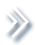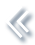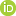####ArchivesReview Articles

## Discussion on the paper “A review of distributed statistical inference”

Junlong Zhao

School of Statistics, Beijing Normal University, Beijing, Chinazhaojunlong928@126.com

Pages 108-110 | Received 11 Nov. 2021, Accepted 20 Nov. 2021, Published online: 16 Dec. 2021,
• Abstract
• References
• Citations

References

• Anderson, T. W. (2003). An introduction to multivariate statistical analysis (3rd ed.). John Wiley & Sons.
• Bai, Z., & Saranadasa, H. (1996). Effect of high dimension: By an example of a two sample problem. Statistica Sinica, 6(2), 311329. https://doi.org/10.1007/s004400050035
• Banerjee, M., Durot, C., & Sen, B. (2019). Divide and conquer in nonstandard problems and the super-efficiency phenomenon. Annals of Statistics, 47(2), 720757. https://doi.org/10.1214/17-AOS1633
• Braverman, M., Garg, A., Ma, T., Nguyen, H. L., & Woodruff, D. P. (2016). Communication lower bounds for statistical estimation problems via a distributed data processing inequality. In Proceedings of the forty-eighth annual ACM symposium on theory of computing (pp. 1011–1020).
• Cai, T. T., & Wei, H. (2020). Distributed Gaussian mean estimation under communication constraints: Optimal rates and communication-efficient algorithms. arXiv:2001.08877.
• Du, B., & Zhao, J. (2021). Hypothesis testing of one sample mean vector in distributed frameworks. arXiv:2110.02588.
• Garg, A., Ma, T., & Nguyen, H. (2014). On communication cost of distributed statistical estimation and dimensionality. Advances in Neural Information Processing Systems, 27, 27262734. https://doi.org/10.1109/hipc.1997.634533
• Lee, J. D., Liu, Q., Sun, Y., & Taylor, J. E. (2017). Communication-efficient sparse regression. Journal of Machine Learning Research, 18(1), 115144. https://doi.org/10.17077/etd.005893
• Li, M., & Zhao, J. (2021). Communication-efficient distributed linear discriminant analysis for binary classification. Statistica Sinica. https://doi.org/10.5705/ss.202020.0374
• Shi, C., Lu, W., & Song, R. (2018). A massive data framework for M-estimators with cubic-rate. Journal of the American Statistical Association, 113(524), 16981709. https://doi.org/10.1080/01621459.2017.1360779 ,
• Srivastava, M. S., & Du, M. (2008). A test for the mean vector with fewer observations than the dimension. Journal of Multivariate Analysis, 99(3), 386402. https://doi.org/10.1016/j.jmva.2006.11.002
• Tian, L., & Gu, Q. (2017). Communication-efficient distributed sparse linear discriminant analysis. In Artificial intelligence and statistics (pp. 1178–1187).
• Volgushev, S., Chao, S. K., & Cheng, G. (2019). Distributed inference for quantile regression processes. Annals of Statistics, 47(3), 16341662. https://doi.org/10.1214/18-AOS1730
• Wang, L., Peng, B., & Li, R. (2015). A high-dimensional nonparametric multivariate test for mean vector. Journal of the American Statistical Association, 110(512), 16581669. https://doi.org/10.1080/01621459.2014.988215
• Zhang, Y., Duchi, J. C., Jordan, M. I., & Wainwright, M. J. (2013). Information-theoretic lower bounds for distributed statistical estimation with communication constraints. In Neural information processing systems (pp. 2328–2336).

To cite this article: Junlong Zhao (2021): Discussion on the paper “A review of distributed
statistical inference”, Statistical Theory and Related Fields, DOI: 10.1080/24754269.2021.2015861